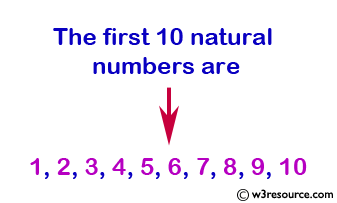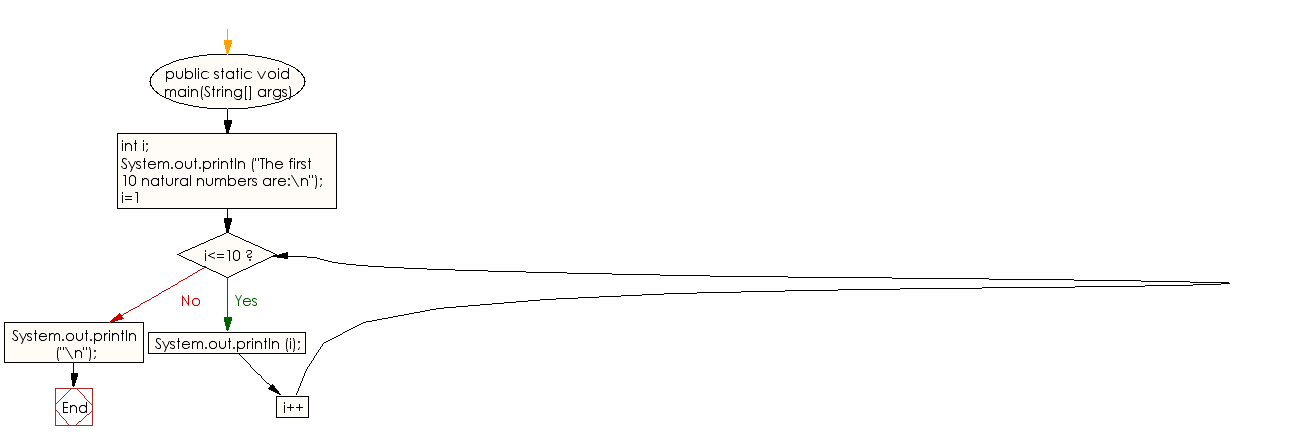﻿ Java - Display the first 10 natural numbers

# Java: Display the first 10 natural numbers

## Java Conditional Statement: Exercise-10 with Solution

Write a Java program to display the first 10 natural numbers.

Pictorial Presentation:Sample Solution:

Java Code:

``````public class Exercise10 {

public static void main(String[] args)
{
int i;
System.out.println ("The first 10 natural numbers are:\n");
for (i=1;i<=10;i++)
{
System.out.println (i);
}
System.out.println ("\n");
}
}
```
```

Sample Output:

```The first 10 natural numbers are:
1
2
3
4
5
6
7
8
9
10
```

Flowchart:Java Code Editor:

What is the difficulty level of this exercise?

Test your Programming skills with w3resource's quiz.

﻿

## Java: Tips of the Day

IsPowerOfTwo

Checks if a value is positive power of two.

To understand how it works let's assume we made a call IsPowerOfTwo(4).

As value is greater than 0, so right side of the && operator will be evaluated.

The result of (~value + 1) is equal to value itself. ~100 + 001 => 011 + 001 => 100. This is equal to value.

The result of (value & value) is value. 100 & 100 => 100.

This will value the expression to true as value is equal to value.

```public static boolean isPowerOfTwo(final int value) {
return value > 0 && ((value & (~value + 1)) == value);
}
```

Ref: https://bit.ly/3sA5d4I

We are closing our Disqus commenting system for some maintenanace issues. You may write to us at reach[at]yahoo[dot]com or visit us at Facebook